# MCQs on Digital Electronics

##### Page 33 of 35. Go to page 1 2 3 4 5 6 7 8 9 10 11 12 13 14 15 16 17 18 19 20 21 22 23 24 25 26 27 28 29 30 31 32 33 34 35
01․ Which is typically the longest: bit,byte,nibble,word?
bit
byte
nibble
word

Bit is the smallest unit in digital representation of any number. Nibble consist of 4-Bit. Byte consist of 8-bit. Word consist of several bytes.

02․ What is the gray code for the binary 101011?
101011
110101
011111
111110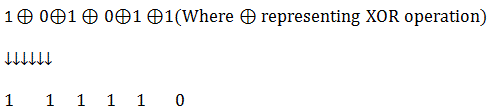03․ A number system with radix 2 is known as
Binary
Octal
Decimal

A number system with radix 2 is known as Binary.

04․ A group of four bits is known as
Bit
Byte
Nibble
Word

A group of four bits is known as nibble.

05․ The binary number 110011.11 is equivalent to decimal number
50.75
51.65
51.75
50.65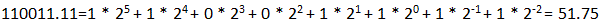06․ The decimal number 0.246 in binary is
0.011011
0.010111
0.001111
0.001101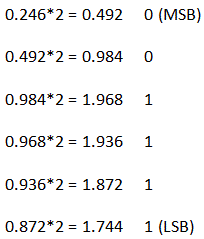07․ The 1’s complement of 11010111 is
00101000
10101000
11101000
10101000

As we know 1’s complement is done by replacing 1 with 0 and 0 with 1 so in this case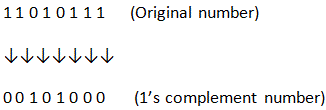08․ Which of the following is 1’s complement representation of -34?
11011110
10100010
01011101
11011101

In 1’s complement representation negative numbers are denoted by 1’s complement of positive number. Binary of +34 is 00100010 -34 will be represented as 1’s complement of (00100010) i.e. (11011101)

09․ Which of the following is 1’s complement representation of +57
00111001
11000110
00000001
01000110

In 1’s complement representation method a positive number is represented by same binary. i.e. (00111001)

10․ What is the 2’s complement of 10110000?
01010000
01001111
10110000
10001110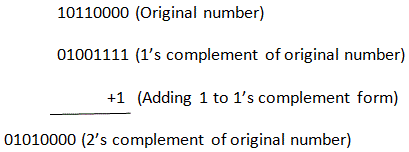<<<3132333435>>>